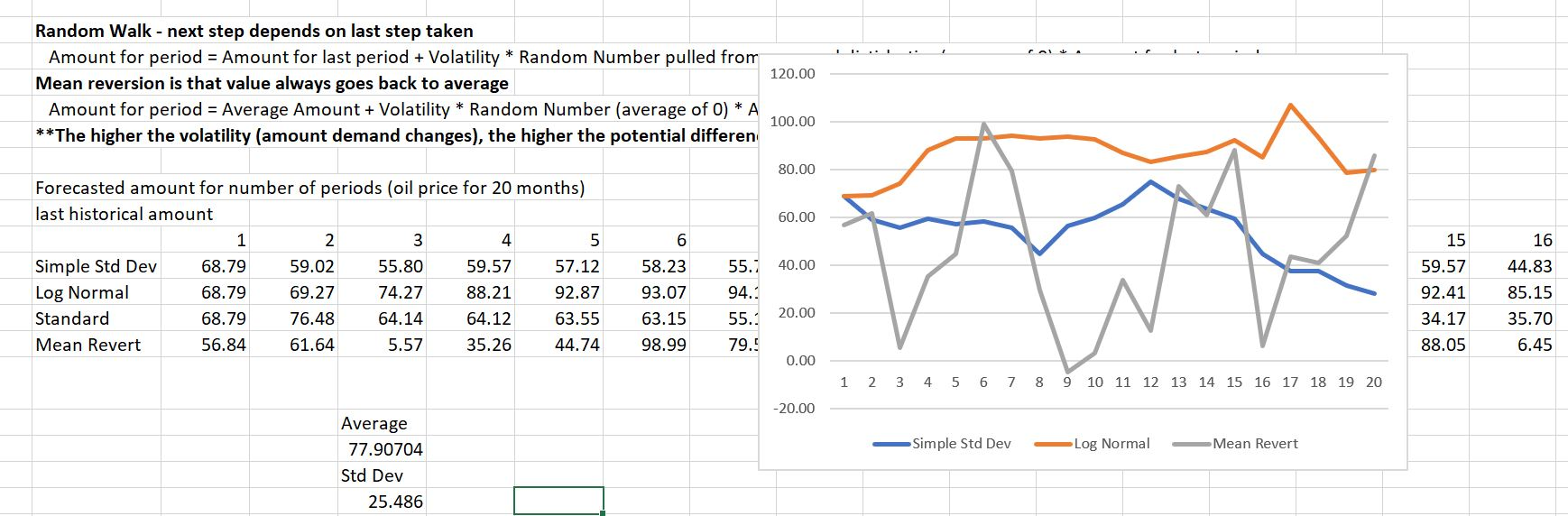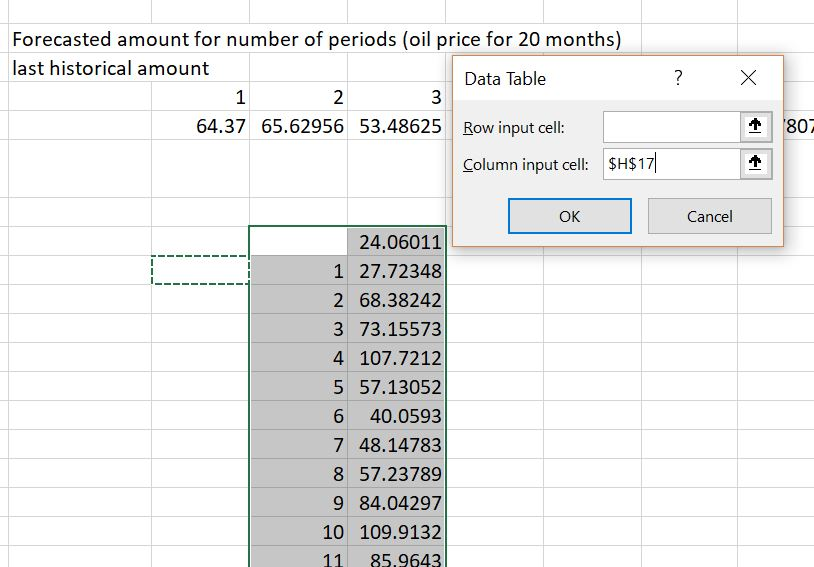# Ease of Adding Monte Carlo Simulation to Financial Models

This page demonstrates how to construct a Monte Carlo simulation assuming you have a model of the system or the process that you want.  The model must have a component for uncertainty that could be volatility multiplied by a random draw from a Monte Carlo simulation.  Three approaches to implementing simulation are demonstrated.  The first method is simply to copy the simulations into multiple rows or columns.  The second method is to use a blank data table with a count of the number of simulations.  The third method is to write a few simple lines of code in a VBA program.

## Why Monte Carlo can be B.S.

When I first began studying Monte Carlo simulation in the early 1990’s I quickly became addicted to the process. You can make beautiful graphs of frequency distributions, show fancy time series equations and directly associate risks with mathematical statistics (i.e. volatility and mean reversion). I have also noticed that academics like to apply Monte Carlo to many problems so they show how smart they are. More recently I have seen that much of the application of Monte Carlo simulation to finance is rubbish. Incorrect measurement of mean reversion can mess things up and many times Monte Carlo does not really measure anything at all.

I do still believe that Monte Carlo can be helpful in certain situations. For example, if you are evaluating how much a cash flow sweep reduces default probability, you can use Monte Carlo simulation. You could measure the default probability with and without a sweep using Monte Carlo simulation. I also find Monte Carlo simulation useful to prove statistical concepts. I did not really believe the Mean Square Error can be used to add up standard deviation, but with simulation this can be demonstrated. Finally, if you want to demonstrate risks associated with holding different portfolios of short-term and long-term debt, the potential risks can be effectively displayed with Monte Carlo Simulation.

## Set-up of Monte Carlo Simulation and Using Simple VBA Program

The following Examples of Models apply that Include Monte Carlo Simulation. These models create time series variable(s) and use a simple macro to apply these in a simulation Framework.  I have made a very simple example that you can download by pressing the button below.  This is the file used in the simple examples.

The file includes a few different ways to compute uncertainty and time series equations (these are discussed in much more detail on subsequent pages). These alternative equations are illustrated in the screenshot below. When you press the F9 button to re-calculate, the graphs change.  Monte Carlo involves taking one of the values (e.g. the value in period 15) and then recording that value for many different scenarios.To record the different values, you can do three things.

The first is to simply copy and past the equation to multiple rows in excel.  There is no big problem with this, in the simple example.  But it the equation is part of a big financial model with many equations, then this not adequate.

The second method uses a  data table to pick a variable.  Say you want to pick something out of a financial model like the IRR that depends on the risk built into the equation. This time, you would not want to repeat the entire model. Instead, you could create a data table that has nothing but 1,000 or 10,000 rows or columns.  Then when you make the data table, it is just like you are pressing the F9 key 1,000 or 10,000 times.  This process that points the column or row input to a blank cell is illustrated below.

The third method uses a little bit of VBA code.  You can create a for, next loop together with the cells function.  All you need to do is use the cells function to record the different scenarios.  The VBA code is illustrated in a separate section below.  The VBA code is very simple and more flexible than the data table method.

## Using a Data Table to Create a Monte Carlo Simulation

Use of a data table to construct a Monte Carlo simulation is illustrated in the screenshot below.  In this case note that the column is a simple list of numbers (it could be from 1 to 10,000).  The number in above and to the left is simple one of the numbers selected from the time series equation (it could be the IRR, the default, the remaining debt at the end of a project etc.)  The column input cell just points to a blank cell anywhere in the sheet (note that it must be the column input cell and not the row input cell).The video below discusses how to set-up a spreadsheet for creating a Monte Carlo simulation.

.

.

## Presenting Monte Carlo Distributions Using the Frequency Distribution

The screenshot below illustrates how to create a frequency distribution from a Monte Carlo Simulation.  On the left-hand side, the long series of numbers created from the Monte Carlo simulation is listed (this could be from the data table or from the VBA code).  From this list you can find the minimum and maximum and then create and increment (you can also use something like the average plus and minus three of four standard deviations).  The increment is the shown in the column starting with 9.69 and increasing by 8.0.  Then you can compute the frequency by using the frequency distribution.  You can select the area next to the increments or bins and then put in a formula for the frequency distribution.  You need to enter the long list of numbers and then the bins.  As this is an array function, press SHIFT, CNTL, ENTER to get the result.## Monte Carlo Exercises

The first two simple exercises begin with a simple Monte Carlo simulation created without a macro below.
The next exercise performs a similar Monte Carlo simulation using Brownian Motion, but uses a simple macro to perform the simulation.
The third exercise includes mean reversion and price boundaries in the Monte Carlo simulation.
The fourth exercise includes correlation among variables in the simulation.

.

.

## Application of Monte Carlo in the Context of Cash Sweeps

7. Cash Sweep and Covenant Analysis Monte Carlo Simulation.xlsm

Commodity Price Model with Monte Carlo Simulation.xlsm

Petrozuata – Finished Simulation.xlsm

1. Run the Monte Carlo simulation for one or more input variables in the cash flow model (e.g. oil prices, gas prices and interest rates). This file allows you to incorporate Monte Carlo simulation with mean reversion, price boundaries, price jumps, correlations and other factors to any input variables
2. Save the Monte Carlo results in a summary file by running the “strip files” macro
3. With the original financial model open and the summary Monte Carlo file open, select the Simulation Template Model

Monte Carlo Comprehensive.zip

Simulation Template.zip

.

.

.